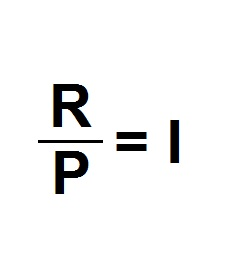Periodic Rate | Propertylogy
Don't Miss

# Periodic Rate

By on August 20, 2018R/P = I

Where

R = Annual interest rate

P = Number of periods

I = Periodic rate

The periodic rate is used to calculate interest rate that has been charged on a loan over a specific period of time.

It is simply calculated by dividing the annual interest with the number of periods.

This rate can then be used to calculate monthly compounding interest on a loan, as well as daily.

For example, if the annual rate is 6% with a monthly period, the monthly periodic rate would be

0.06/12 = 0.005 or 0.5%

We can then use this to calculate the compounding interest

On a \$100,000 compounding interest for 7 months would be

(1 + 0.005)7 = 1.0355

Then, 1.0355 x \$100,000 = \$103,550

The dollar amount after 7 months would be \$103,550.

Send this to a friend